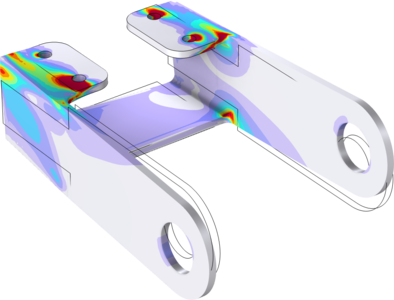# Application Gallery

#### Bracket — Transient Analysis

Application ID: 10314

The bracket models are used as an introduction to structural mechanics modeling with the Structural Mechanics Module.

The following features are introduced:

• The fundamentals: static linear analysis
• Parametric study
• Including initial strain
• Modeling thermal expansion
• Adding a rigid connector
• Adding spring conditions
• Modeling with the Shell interface
• Transient analysis
• Eigenfrequency analysis
• Prestressed eigenfrequency analysis
• Frequency response analysis
• Prestressed frequency response analysis
• A geometric nonlinear problem: linear buckling modeling
• Modeling contact problems
• Analyzing prestressed bolts with contact
• Presenting results from shell element analyses in 3DThis model example illustrates applications of this type that would nominally be built using the following products: